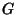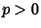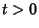## Abhyankar's Conjecture

For a Finite Group, letbe the Subgroup generated by all the Sylow p-Subgroup of. Ifis a projective curve in characteristic, and if, ...,are points of(for), then a Necessary and Sufficient condition thatoccur as the Galois Group of a finite coveringof, branched only at the points, ...,, is that the Quotient Grouphasgenerators.

Raynaud (1994) solved the Abhyankar problem in the crucial case of the affine line (i.e., the projective line with a point deleted), and Harbater (1994) proved the full Abhyankar conjecture by building upon this special solution.

References

Abhyankar, S. ``Coverings of Algebraic Curves.'' Amer. J. Math. 79, 825-856, 1957.

Harbater, D. ``Abhyankar's Conjecture on Galois Groups Over Curves.'' Invent. Math. 117, 1-25, 1994.

Raynaud, M. ``Revêtements de la droite affine en caractéristiqueet conjecture d'Abhyankar.'' Invent. Math. 116, 425-462, 1994.